# iFit: Mathematical operators

1. A reminder on axes, monitors and signal errors
2. Unary operators
3. Binary operators
4. Statistics, fitting and peak searching, segmentation (k-means)
5. Projection, integration and sum
6. Cutting, Merging and combining data sets
7. Appending and slicing data sets
8. Creating arrays of objects
9. Interpolation, smoothing, intersection and union

In this documentation, we review most of the mathematical operations that can be applied on iData objects. Separate help pages are available for data import, export and fitting. A full list is available in the methods page.

### A reminder on axes, monitors and signal errors

The iData objects have been designed keeping in mind that any measurement Signal is recorded as a function of Axes (e.g. time, energy, ...) and corresponds to a measurement quantity (e.g. flux, integrated time for the measurement, ...), which we label as a Monitor. In addition, the Signal is attached to an uncertainty Error, which provides a measurement of the accuracy of the Signal. You may get more details about these definitions in the iData object description page. Remember that axis of rank 1 is usually associated to Y (vertical, along rows), whereas axis of rank 2 is associated to X (horizontal, along columns) except for 1D object where X is rank 1.

To get the signal and axes values, use:
```>> a = iData([ ifitpath 'Data/ILL_IN6.dat' ]);>> a{0}; % or getaxis(a, 0) to get the Signal (0-rank)>> a{1}; % or getaxis(a, 1) to get the 1-st rank axis, that is 'x' for vector-type data, and 'y' for 2D+>> a{2}; % or getaxis(a, 2) to get the 2-nd rank axis, that is 'x' for 2D+ data sets
```
In case the axis definitions is not what you want, you can re-assign them using the setaxis method (refer to the iData object documentation).

The way all of these are used is listed below:
• All mathematical methods propagate the Error together with the Signal, assuming Error distributions are Gaussian. The sections below detail the way Errors are propagated.
• Binary operators use the Monitor value to weight the respective ratio when performing the operation. The Monitor value is also affected by Mathematical operations that renormalize the Signal. The Monitor is usually a value larger than 1. When set to 0 (or empty), no weighting is used.
• The Axes are used in binary operators to determine intersection of Signal ranges. A rebinning of objects is automatically performed when the Axes sampling do not match.

### Unary operators

All unary operators apply on a single object, element-by-element. The Error for an operator f is usually is

new Error = Error.f'(Signal).

In the cases where the Error can not be determined analytically, and approximation is obtained using

new Error = f(Signal + ½Error) - f(Signal - ½Error).

Warning: all the operators work on the normalised Signal/Monitor and Error/Monitor.

 Operator new Signal new Error new Monitor Comments abs | Signal | Absolute value acos cos-1(Signal) Error/(√(1-Signal²)) Arc cosine. Signal should be [-1:1] asin sin-1(Signal) Error/(√(1-Signal²)) Arc sine. Signal should be [-1:1] atan tan-1(Signal) Error/(1+Signal²) Arc tangent. cos cos(Signal) -Error*sin(Signal) Cosine. Signal is in [rad] sin sin(Signal) Error*cos(Signal) Sine. Signal is in [rad] tan tan(Signal) Error/cos²(Signal) Tangent. Signal is in [rad] cosh, sinh, tanh Hyperbolic trigonometric functions acosh, asinh, atanh Inverse hyperbolic trigonometric functions exp eSignal Error*eSignal Exponential log ln(Signal) Error/Signal Logarithm (Neperian, base 2) log10 log10(Signal) Error/(ln(10)*Signal) Logarithm (base 10) sqrt √Signal Error/(2√Signal) √Monitor Square root ctranspose and ' Signal' Error' Monitor' Complex conjugate transpose transpose and .' Signal.' Error' Monitor' Non conjugate transpose. permute permute(Signal) permute(Error) permute(Monitor) dimensionality permutation (generalized transposition) floor floor(Signal) floor(Error) Round towards -∞ Make it integer. ceil ceil(Signal) ceil(Error) Round towards +∞ Make it integer. round round(Signal) round(Error) Round towards nearest integer. sign sign(Signal) 0 Sign of Signal elements. uminus and - -Signal Opposite imag ℑ(Signal) Imaginary part real ℜ(Signal) Real part fft FFT(Signal) FFT(Error) Fourier transform (see below) ifft FFT-1(Signal) FFT-1(Error) inverse Fourier transform cwt Wavelet(Signal) Continuous wavelet transform (see below) del2 ∇2(Signal) ∇2(Error) Laplacian ∑i ∂2Signal/∂2Xi gradient ∇(Signal) ∇(Error) Gradient (partial derivatives). See also the 'jacobian' operator for coordinate changes. diff ∇1(Signal) ∇1(Error) Gradient on 1st axis (rows) sum ∑(Signal) √∑(Error²) Sum of the Signal elements (see below) prod ∏(Signal) ∏(Signal+Error/2)-∏(Signal-Error/2) Product of the Signal elements trapz ∫(Signal) √∫(Error²) Trapezoidal integration cumsum ∫0x(Signal) √∫0x(Error²) Primitive smooth Smoothed(Signal) Smoothed(Error) Robust spline smoothing based on cosine transform kmeans Partition indices 0 Data segmentation (k-means) pca Principal component coordinates 0 Principal component analysis resize Resized signal resized Error resized Monitor fast resize with interpolation reducevolume smaller Signal - - data set reduction sort sorted Signal - - sorted data set along a dimension
Unary operators for iData objects

Here is an example using unary operators:
`>> a = iData([ ifitpath 'Data/ILL_IN6.dat' ]);>> b = [ log(a) floor(a) sqrt(a) ]b =  array [1  3] iData object:Index     [Tag]     [Dimension]                           [Title] [Last command]          [Label]    1  tp362134     [340  1024] 'File ILL_IN6.dat ILL Data (n...' tp362134=log(tp36213...      2  tp362140     [340  1024] 'File ILL_IN6.dat ILL Data (n...' tp362140=floor(tp362...      3  tp362145     [340  1024] 'File ILL_IN6.dat ILL Data (n...' tp362145=sqrt(tp3621...  `
The 1/Signal is obtained with the binary operator division. The sqr operator is a short cut for power 2.

#### Fourier transform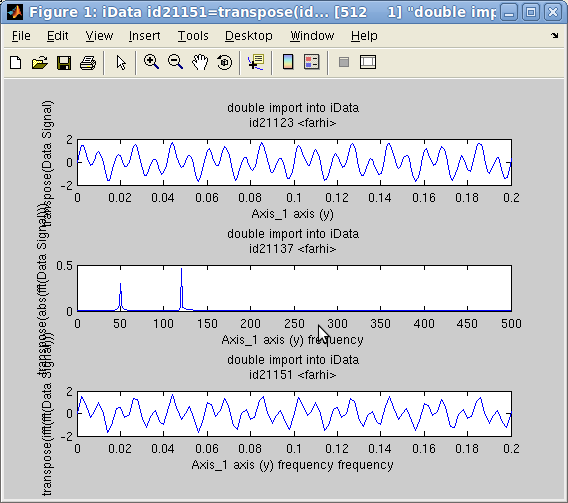The Fourier Transform operators fft and 'inverse' ifft perform a Discrete FFT computation from an N-dimensional object. The axes are converted to frequency/reciprocal axes.
In the following example, we create an object which Signal is the sum of 2 sine functions with frequencies 50 and 120 Hz. Then we compute its FFT, and again its inverse FFT.
`>> t=linspace(0,1,1000);>> a = iData(t,0.7*sin(2*pi*50*t)+sin(2*pi*120*t)+0.05*randn(size(t)));>> c=fft(a); d=ifft(c); subplot([ a abs(c) d ]);`
The plot on the side shows that the two sine components are identified (the FFT is shown in modulus), and the FFT-1(FFT) is indeed the initial signal (within the computation approximations).

The convolution operator, which uses FFT multiplication, is detailed below. The frequency power-spectrum is the square of the modulus of the FFT, sqr(abs(FFT)).

#### Wavelet transform

Going further than the FFT, the cwt method computes the continuous wavelet transform of a data set. It corresponds to the decomposition of the signal along a wavelet basis function set, that is a multi-scale analysis of the signal along an axis. In the following example, we analyse a 2D powder neutron diffractogram with the cwt and the slice methods.
`>> a=iData([ ifitpath 'Data/Diff_BananaPSD_1314088587.th_y');>> w=cwt(a, 2);	% the cwt along the axis rank 2 (scattering angle)>> plot(a); slice(log(abs(w).^2))	% plot the initial data set and its cwt power spectrum`
The resulting plot shows the sharp features (peaks) for small 'periods', and the broad features (background) for large periods. The period axis is here shown in log10 scale of the initial axis (degrees) and corresponds to the width of the wavelet.
Reference: Grinsted, A., Moore, J.C., Jevrejeva, S. (2004) Application of the cross wavelet transform and wavelet coherence to geophysical time series, Nonlin. Processes Geophys., 11, 561–566, doi:10.5194/npg-11-561-2004.

#### Derivatives and variable change (Jacobian)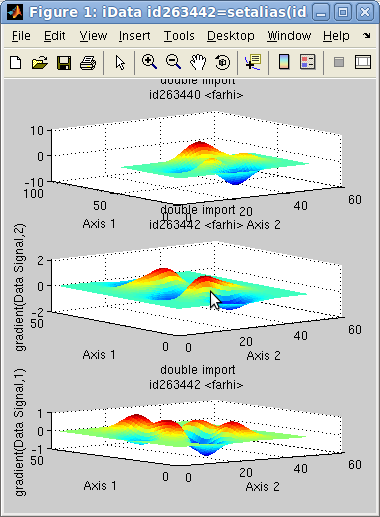The gradient operator computes the partial derivatives ∇ of an object. (see Gradient definition)

∇a = (∂a/∂Y ∂a/∂X ∂a/∂Z ...)

The resulting objects are sorted by axis rank, that is partial(X) is 2nd, partial(Y) is 1st, partial(Z) is 3rd, etc. In case the axis sampling/binning is not regular, a re-binning is automatically performed. For 1D objects, the gradient is the derivative. In the following example, we compute the 2D gradient of an object, and plot them.
`>> a=iData(peaks);>> g=gradient(a); subplot([ a g(1) g(2) ]);`
The diff method computes the gradient for the rows only (1st axis).
The del2 operator computes the Laplacian Δ=∇2 of an object (see Laplacian definition)

2a = ∑i (∂2a/∂Xi2)

which is a measure of the curvature of the object. In this sense, for an N dimension object, it schematically returns, with the del2 standard Matlab function, 2N*del2(Signal). In case the axis sampling/binning is not regular, a rebinning is automatically performed.

Finally the jacobian operator (see Jacobian definition) enables to perform a variable change in the representation of the Signal. This corresponds to a new axis definition with consecutive correction of the Signal so that the integral value is retained between the different space representations.

Suppose we want to perform a coordinate change from the axis system X={X1, ... Xn} to a new space Y={Y1, ...Yn} where n is the dimensionality of the object a Signal. Then the Jacobian of the object is:

J(a, X, Y) = [ dYi/dXi ]ij

The new object b infinitesimal element (pixel/bin) in the new representation which ensures ∫ a dX = ∫ b dY is such that:

b  = a | dY/dX |

which requires to estimate the Jacobian determinant for each pixel/bin in the initial object a. As the Signal holds a single scalar value in each bin, J is the division of the gradient of Y over the gradient of X for the whole Signal.

### Binary operators

The binary operators take two iData objects, and perform a relational operator, element-by-element. In the following we use the notations:
• s1, e1 and m1 are the Signal, Error and Monitor of the 1st object, resp.
• s2, e2 and m2 are the Signal, Error and Monitor of the 2nd object, resp.
• s3, e3 and m3 are the Signal, Error and Monitor of the resulting object, resp.
When not defined, the Monitor is set to 1, and the Error is set to √Signal. When the Monitor is set to 0, the Signal is not divided by it. Then the binary operator result has the other object's Monitor (which may also be 0).

 Operator Signal (s3) Error (e3) Monitor (m3) Description plus and + m3*(s1/m1+s2/m2) √[(e1/m1)²+(e2/m2)²] m1+m2 addition minus and - m3*(s1/m1 - s2/m2) √[(e1/m1)²+(e2/m2)²] m1+m2 subtraction times and .* s1 * s2 s3*[(e1/s1)²+(e2/s2)²] m1*m2 multiplication (element by element). When object orientations are complementary (orthogonal), the final object is built with dimensionality expansion. mtimes and * s1 x s2 e1 x e2 m1 x m2 matrix multiplication rdivide and ./ m2²*(s1/s2) s3*[(e1/s1)²+(e2/s2)²] m1*m2 division (element by element). When object orientations are complementary (orthogonal), the final object is built with dimensionality expansion. mrdivide and / see above see above see above matrix division: currently equivalent to the divide operator combine and \ s1+s2 √[e1²+e2²] m1+m2 add Signals and Monitors independently (see below). Event lists objects are catenated. power and .^ m3*(s1/m1)(s2/m2) s3*[s2*e1/s1+e2*ln(s1)] m1m2 exponentiation. When object orientations are complementary (orthogonal), the final object is built with dimensionality expansion. comparisons: lt, le, gt, ge, ne, eq comparison(s1, s2) √[(e1/m1)²+(e2/m2)²]/[s1/m1+s2/m2] 1 binary comparisons conv FFT-1(FFT(s1)*FFT(s2)) s3*[(e1/s1)²+(e2/s2)²] (this may be inappropriate) m1*m2 convolution (see below) deconv iterative deconvolution (see below) xcorr FFT-1(FFT(s1)*FFT*(s2)) s3*[(e1/s1)²+(e2/s2)²] (this may be inappropriate) m1*m2 cross correlation (see below) interp s1 onto s2 axes e1 onto s2 axes m1 onto s2 axes interpolation (linear is default, see below) corrcoef cov(s1,s2)/σ(s1)σ(s2) in [-1:1] Pearson correlation coefficient
Binary operators for iData objects

All these operations find intersection ∩ between objects (when axes are e.g. partly overlapping), rebin objects when axes have a different sampling, and then perform the mathematical operation. In case some objects have a lower dimensionality, they are extended along missing dimensions (by mere duplication) to match the higher dimensionality, so that the operation can be performed.The following example adds a 2D data set with a 1D data set, which is extended. The intersection selects only the positive axis values from the 1D object, here in [0 5]. As the 2D object has no default Monitor (set to 1), we set it to 1/10 of the corresponding mean value in the 1D object (if not done so the IN6 data is under weighted compared with the IN20 data for which Monitor is high). The magenta and blue arrows indicate how the data is added, and the 1D data set extended along the Axis 2. as shown with the double arrow.
`>> a = iData([ ifitpath 'Data/ILL_IN6.dat' ]);>> b = iData([ ifitpath 'Data/ILL_IN20.dat' ]); >> set(a,'Monitor', mean(b.Monitor)/10);>> c = a+b;>> subplot([ log(a) b log(c) ] ,'tight')`
Warning: It is important to check that the axes and Monitor values are consistent when performing binary operations, otherwise the intersection may be empty, or the corresponding Signal weighting may be wrong.

The combine operation will be detailed in the section 'Merging and combining data sets' below. In particular, the difference between the summation and the combination will be detailed there.

All binary operators can also be used between one iData object and a file name, in which case the corresponding data file is first imported before the operation is performed.
`>> a = iData([ ifitpath 'Data/ILL_IN6.dat' ]);>> c = a+[ ifitpath 'Data/ILL_IN20.dat' ];`

#### Binary operators between objects of different type (Data sets, Models, ...)

When a binary operation is requested between a Data set (iData) and/or a Model (iFunc) or other argument type, the result usually depends on the nature of the first argument, as detailed in the following table.

 First argument Second argument Result Example iData iFunc iData. The Model (2nd arg) is evaluated using the Data set (1st arg) axes, and the operator is then applied. iData(1:10)+gauss iData iData iData iData(peaks)+iData(rand(25)) iData char iData. The 2nd argument is considered to be a file name or URL, and loaded before the operator is applied. iData(peaks) + [ ifitpath 'Data/ILL_IN6.dat' ] char iData iData. The 1st argument is considered to be a file name or URL, and loaded before the operator is applied. [ ifitpath 'Data/ILL_IN6.dat' ] + iData(peaks) iData scalar/vector/matrix iData. The 2nd argument should be scalar, or have compatible dimensions with the Data set. iData(peaks)+ rand(49)iData(peaks)+ 2 scalar/vector/matrix iData iData. The 1st argument should be scalar, or have compatible dimensions with the Data set. rand(49)+iData(peaks)2+iData(peaks) iFunc iData iFunc. The Data set (2nd arg) is stored in the UserData property of the Model (1st arg), and is dynamically interpolated on the Model axes during evaluation. gauss+iData(1:10) iFunc iFunc iFunc gauss+lorz iFunc scalar/vector/matrix iFunc. The 2nd argument should be scalar, or have compatible dimensions with the Model axes. gauss+2 scalar/vector/matrix iFunc iFunc. The 1st argument should be scalar, or have compatible dimensions with the Model axes. 2+gauss iFunc char iFunc. The specified string is directly concatenated 'as is' with the Model expression. The string may refer to global variables. gauss+'2' char iFunc iFunc. The specified string is directly concatenated 'as is' with the Model expression. The string may refer to global variables. '2'+gauss
Binary operator behaviour between mixed objects

#### Convolution/correlation

The convolution operator conv uses a FFT method to compute the convolution of one iData with anything else. In the background it uses the fconv and fconvn function (see Models), which can be called directly when building fit functions.

The convolution operator can be given additional options in order to control the accuracy and the size of the returned object
`>> c = conv(a,b);		% returned convoluted object with size which is size(a)+size(b)+1>> c = conv(a,b, 'same');	% returned convoluted object with size which is size(a)>> c = conv(a,b, 'valid');	% returned convoluted object with size which is size(a)-size(b)+1>> c = conv(a,b, 'pad');	% pads 'a' with starting/ending values to minimize border effects>> c = conv(a,b, 'center');	% centers 'b' so that convolution does not shift 'a' axes>> c = conv(a,b, 'norm');	% normalizes 'b' so that convolution does not change 'a' integral>> c = conv(a,b, 'background');	% subtracts minimal value in 'b' so that convolution does not change 'a' integral>> c = conv(a,b, 'deconv');	% deconvolution, but very sensitive to noise (use with caution)`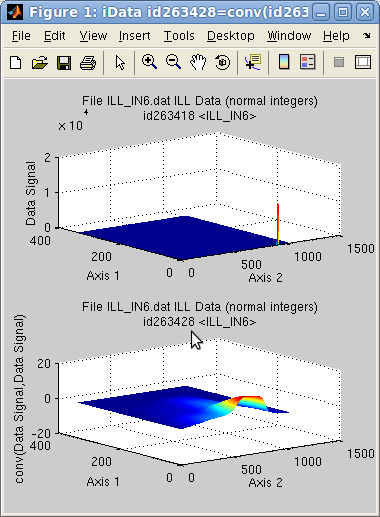When the 1st object has to be convoluted with a response function (e.g. instrument resolution function), the usual options to use should be:
`>> c = conv(a,b, 'same pad background center norm');>> c = convn(a,b);	% same as above in a shorter call`
To perform an auto-convolution, just use:
`>> a = iData([ ifitpath 'Data/ILL_IN6.dat' ]);>> c = convn(a,a);`
When the second argument 'b' is given as a scalar, a Gaussian with that width is built.
`>> c = convn(a, 3)	% convolution of an iData with a normalized Gaussian of width 3`
This is very useful when a measurement has to be corrected with the response of the probe (instrument). In this case, a fit of the data may be carried out as:
`>> global b;			% holds the convolution function as a single vector/matrix or iData object>> h = convn(gauss, 'double(b)');	% creates a fit function with convolution>> h.Constraint= 'global b'; 	% declares b as an external global variable>> p = fits(a, h);`
where the vector/matrix 'b' holds the response function (filter) with the same axis binning as the object 'a'. A way to force the right binning is to use:
`>> c = interp(b, a);`
if the axes of 'b' match the ones from 'a' in range (overlap).
An other example of fit function with convolution is detailed in the Models help page.

The deconv operator performs a deconvolution, using either FFT's or with an iterative method.

Similarly, the xcorr operator computes the cross correlation of two signals. The auto-correlation is simply xcorr(a).

Last, the corrcoef method returns the Pearson product-moment correlation coefficient, which measures the similarity between two data sets (1=equal, 0=non correlated, -1=anti-correlated).

Warning: The accuracy of the conv and xcorr operators depends on the axis sampling. A coarse axis sampling (that is a reduced number of points under a peak, e.g. less than 5) must be avoided. A rebinning of the objects using e.g. interp(objects, 5) may solve such issue.

#### Comparisons

The usual comparison binary operators
• greater: gt >
• greater or equal: ge >=
• lower: lt <
• lower or equal: le <=
• equal: eq ==
• not equal: ne ~=
are also implemented. They compares the Signals, taking the Monitor weighting into account. The associated error bar is normalized to the mean signal.

### Statistics, fitting and peak searching, segmentation (k-means)

A few methods have been written to analyze the Signal from objects. This includes peak searching, first and second moment distributions, and model fitting.

#### Statistics

The usual min, max, mean, and median methods can be applied onto iData objects, and return the corresponding statistics for the Signal. The operation is performed for one given axis/dimension, e.g. along y, x, z ...
`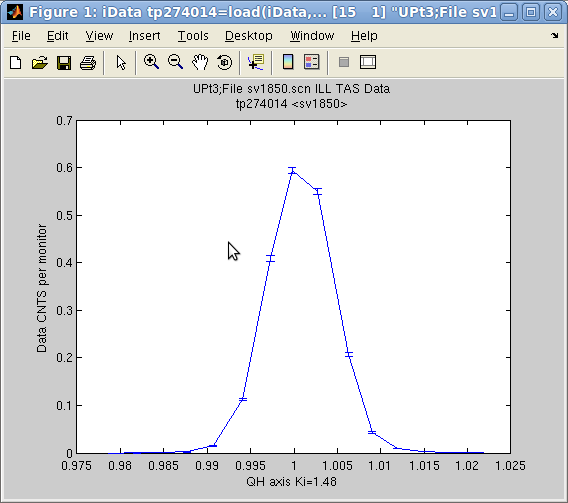`
The std method provides an easy way to determine Gaussian widths of Signal distributions along axes. It returns the half width (2nd moment) and center (1st moment) of the Signal distribution.
`>> a = iData([ ifitpath 'Data/sv1850.scn' ]);>> [w,x]=std(a)w =    0.0036x =    1.0007>> [ min(a) max(a) median(a) mean(a) ]ans =            0         7387          119       1630.7`
indicates that the Signal is distributed in 1.0007 ± 0.0036, and gives min, max, ... As a comparison, the fit to a Gaussian function returns
`>> fits(a)   'Amplitude'    'Centre'    'HalfWidth'    'Background'      0.67857       1.0008    0.0034641   0.00024067`
which is about the same as the 1st and 2nd moment estimates. Here, the Amplitude fit parameter is the one for Signal/Monitor, which is indeed different from the value returned by max when Monitor is not 1. Use setalias(object, 'Monitor',1) to get similar values from fits and max in this example.

Note: If you get an imaginary width from std you may use a negative dimension specification, e.g. std(a, -1), in order to subtract automatically the minimum value from the object, and get a better estimate.

To get the width and centroid of a multi-dimensional object, you can use an array of dimension specification, e.g.:
`>> a = iData(peaks);>> [w, x] = std(a, -(1:ndims(a))); % we subtract the minimum value (negative dimension spec.)`
It is also possible to compute with the corrcoef method the correlation coefficient between an object and something else, e.g. a vector, an iFunc model or a single vector of same length as the object signal.

These operators apply iteratively on object arrays. The mean and median then point to the combine operator, the min and max return the minimum and maximum along all objects.

#### Peak searching and model fitting

The peaks method makes an automatic analysis of a vectorial Signal (that is projected along one given dimension) of an object, and determines the underlying baseline (background), apparent peak position and width, based on a shifting Gaussian estimate [see Slavic, Nucl. Inst. Meth. 112 (1973) 253 ; M. Morhac, Nucl. Inst. Meth. A 600 (2009) 478].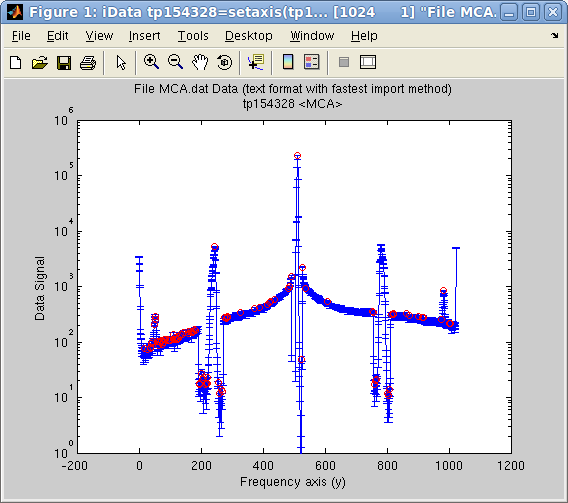This method works remarkably for signals with sharp peaks. The following example displays the automatically found peaks on top of the object view:
`>> a = iData([ ifitpath 'Data/MCA.dat' ]);>> setalias(a,'Signal','Data.Counts(:,2)');>> [half_width, center, amplitude, baseline]=peaks(a);>> plot(a); hold on; >> plot(center,amplitude,'ro'); set(gca, 'yscale', 'log')`
The first dimension (projection along rows, rank 1) is selected by default for the analysis, but it may be specified as the second argument. For broader peaks, a 3rd argument can be used to specify the length m of the moving window used for the analysis, which should typically be about the number of point forming a peak (default is about 5).

As opposed to the std method shown above, which only provides one Gaussian estimate, the peaks method can handle as many peaks as necessary, and is very fast. It also works with iData object arrays. This method is also used as a basis to determine some starting parameters for most fit functions (see the Fitting and the Optimizers pages).

In the case where a better peak estimate is required, or any other advanced model parameter determination, the fits method can be used. This latter makes use of the eval method, which evaluates a model function onto the object axes.

#### Data segmentation (partitioning/clustering, k-means)

It is possible to perform a statistical analysis of a data set in order to classify it into a number of categories. This is called segmentation, and a usual method to achieve it is the k-means algorithm. The corresponding iData method is kmeans :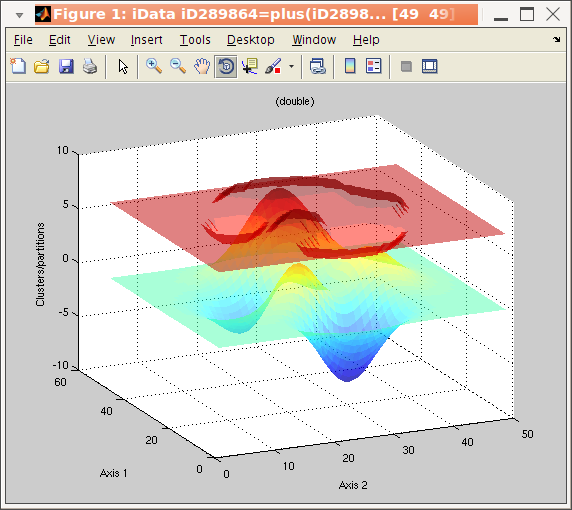`>> a = iData(peaks);>> b = kmeans(a, 3);	% request segmentation in 3 groups>> plot(a, b+5);	% overlay data set and segmentation result`
The wavelet transform cwt may also be used to perform a peak analysis, separating sharp features from broad ones (see above).

The principal component analysis (PCA) methodology consists in finding similarities between data sets. Groups of 'close' data sets can then be defined. The corresponding iData method is pca .

### Projection, integration and sum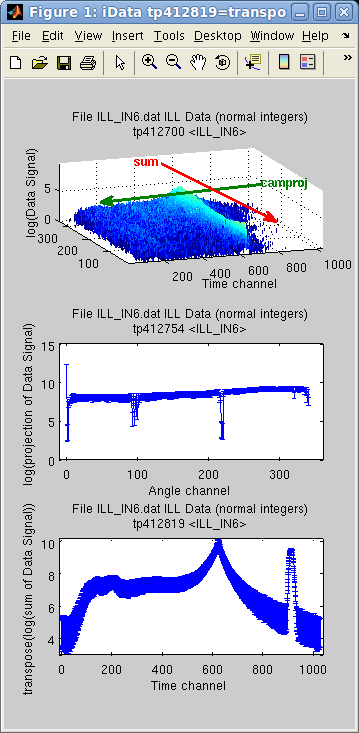There are a number of methods used to extract projected and summed objects. Technically, the projection on one axis is the sum along all other axis.

sum(a, rank) = ∑ Signal(rank axis index)

camproj(a, rank) = ∑ Signal(all axes except rank)

In the following example, the imported object is assigned two axes, and we then display the difference between the sum and the projection with camproj, onto the 1st axis (y). Of course this can be changed by specifying explicitly the desired axis rank used for operation as the second argument.
`>> a = iData([ ifitpath 'Data/ILL_IN6.dat' ]);>> xlabel(a, 'Time channel'); % 2nd axis>> ylabel(a, 'Angle channel');% 1st axis>> subplot([ log(a) log(camproj(a)) log(sum(a)) ],'tight');`
The camproj method always returns a 1D result (or a single value). The sum reduces the dimensionality of the object by the number of axes summed. Similarly, the prod method compute the multiplication along a given axis:

prod(a, rank) = ∏ Signal(rank axis index)

The summation with the sum method does not take into account the axes values. In the case a proper integration is needed, the trapz method can be used for integration using the trapezoidal approximation on the axis values and step dx

trapz(a) = ∫ Signal(rank axis) dx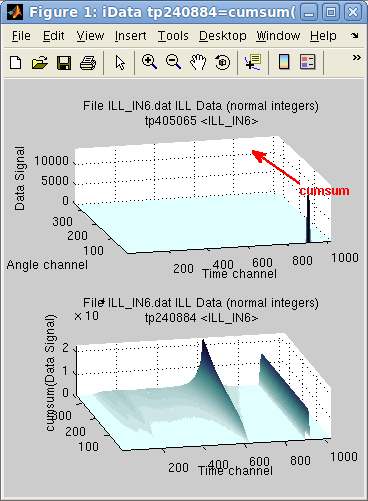which will reduce the dimensionality, and be roughly equivalent to sum when dx=1.

Last, the accumulated versions of sum, trapz and prod, cumsum, cumtrapz and cumprod, return objects of same dimensionality for which the operation is performed gradually along the chosen axis. The last slice along the axis contains the sum and prod results.

In the following example, the sum along the first dimension (y=Angle channel) reveals that an intense peak exists in first angle channel, resulting in a constant line (right side of the sum), whereas the elastic line, is gradually summed over all angle channels, showing inelastic contribution on its shoulders.
`>> subplot([ (a) cumsum(a) ] ,'tight')`
The cumtrapz is the primitive of the Signal, which is equal to cumsum when the axis step is dx=1.

The norm method computes the norm-2 of the object Signal, that is

norm(a) = ½ ∑ |Signal|²

The camproj can also be used with dim='radial' in order to integrate a data set radially. This integration can also be coupled with a more general Cartesian to spherical coordinate transformation with cart2sph :`>> a = iData([ ifitpath 'Data/*.cbf' ]);>> b = camproj(a,'radial'); % returns a distribution to be transformed into an histogram>> c = cart2sph(a);>> subplot(log(a), hist(b,500), log(c))`
To efficiently and quickly reduce the dimensionality of an object, we also recommend the resize method by specifying a singleton dimension:
`>> b = iData(flow);		% is 3D>> resize(b, [25 50 1]);	% makes a 2D object from a 3D one`
Then use squeeze(b) to remove any remaining singleton dimension.

### Cutting, Merging and combining data sets

#### Cutting and selecting portions of a data set

A way to select a subset of an object from its axis rang is to use xlim, ylim, zlim, clim for respectively 2nd (X is 1st for 1D objects), 1st, 3rd and 4th dimensions. It is also possible to directly use find:
`>> a = iData([ ifitpath 'Data/ILL_IN6.dat' ]);>> b = xlim(a, [100 200]);					% selects the object with X axis ranging from 100 to 200>> b = a(:, find(  100 <= a{2} & a{2} <= 200 ));				% same as above: X is rank 2(columns)>> c = a(find(50 <= a{1} & a{1} <= 150),find(100 <= a{2} & a{2} <= 200));	% cuts along X(rank2) and Y axes(rank1, rows)`
`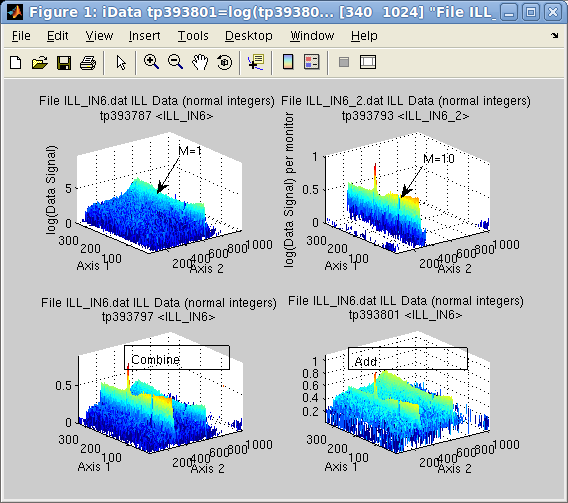`

#### The difference between addition and combination

The combine operation performs an addition of the Signal and the Monitor. This is of great use when merging results from similar experiments, possibly performed in slightly different configurations (e.g. counting time, statistics, ...). This means that combining two data sets is usually more complex than just adding the two Signals, especially when axes are not exactly the same and Monitors are not equivalent.

Suppose we perform a measurement providing a Signal S1 for a given acquisition time M1 (Monitor). The data to be used in mathematical operations is S1/M1. Then we repeat that same measurement, providing Signal S2 and Monitor M2. If, for this example, S2 and M2 are similar to S1 and M1, then the combination of the two data sets should produce a similar signal as any of the two measurements, but with better statistics. But the addition should produce about twice any of the measurements. In short the rules for the operations are:
• combination: (S1+S2) over monitor (M1+M2)
• addition (sum): (M1+M2)*(S1/M1+S2/M2) over monitor(M1+M2)
as listed in the binary operators Table. The addition performs the operation per Monitor value (weighted), whereas the combine operation adds raw Signals and Monitors (unweighted). Changing the Monitor of the objects affects the relative weight used for the summation during the addition operation, but not the combine operation.

When one of the Monitors is set to 0, the Signal is left as is. In the case where the two objects have a 0 Monitor, the addition results simply in the sum of the Signals.

Suppose the first object is the result of a measurement during 1 minute (liquid, M1=1), whereas the second lasted 10 minutes. This means that per Monitor value, the second signal (Vanadium,, M2=10) is much lower than the first one (see upper right signal in subplot aside, compared with upper left) but has a stronger statistical weight.
`>> a = iData([ ifitpath 'Data/ILL_IN6*.dat' ]);>> a(1)=setalias(a(1),'Monitor', 1);>> a(2)=setalias(a(2),'Monitor', 10);>> b=combine(a)             % combine/merge>> c=a(1)+a(2);             % addition>> subplot([ log(a) log(b) log(c) ], 'tight');`
The combined Monitor is M=11, which means that the first data set will appear much lower than initially (the initial Monitor was M1=1) in the combined data set. However, for the add operation, it appears much higher, as each Signal/Monitor is added separately.

The '\' operator is used as a shortcut for combine, so that combine(a,b) is the same as a\b.

#### Gluing an object content inside an other one

As a complement to addition and merging, it is also possible to glue an object 'a' content inside an other one 'b'. In this case, the overlapping region from 'b' is fully replaced by the corresponding one in 'a', without addition/merge. The syntax is
`>> b(1) = a;`

### Appending and slicing data sets

An other way to make use of a set of data from a series of acquisitions is to append them one after the other. This operation, also referred as concatenation, may be performed along an existing dimension (one after the other), or along a new dimension (side by side), which then increases the object dimensionality. The operator used here is cat.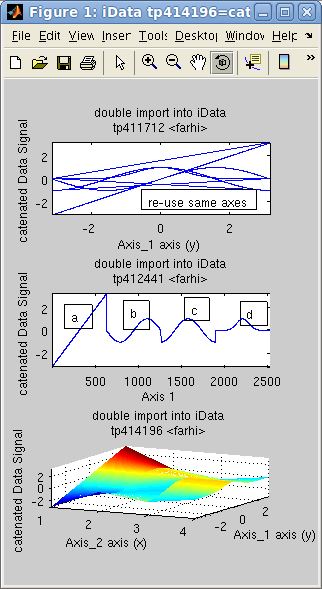In the following example, the object d is the concatenation of 4 objects [a b c d], sequentially. The concatenation of the 4 axes in 'd' is the repetition of the initial axis. We then create an 'f' object for which the axis is reset to the default, showing indeed the concatenation of objects. Last, we assemble the same data set side by side onto a new dimension, creating a surface 'g'.
`>> x=-pi:0.01:pi; a=iData(x,x); >> a.Error=0;                         % replace default Error=sqrt(Signal) by no-error.>> b=sin(a); c=cos(a); d=exp(-a.*a);  % create new objects by applying operator on the initial linear one>> e=cat(1, [a b c d ]);              % catenate along all X axes, result is a single vector>> f=copyobj(e);>> rmaxis(f,1);                       % reset object axis to the default>> g=cat(2, [a b c d]);               % append objects side-by-side onto new dimension>> subplot([e f g],'tight')           % plot all>> h=dog(2, g);                       % back to [a b c d] with 'dog'`Similarly to the cat, the dog operator splits an object into slices along a given dimension, returning an array with as many elements as the length of this dimension. This is the opposite to the cat (of course !).

To extract a slice out of an object, the usual array indices selection is effective:
`>> a = iData(peaks);>> b = a(10, :);		% row 10>> c = a([ 5 7 ], 1:2:end);	% rows 5 and 7, one every 2 columns`

#### Graphical user interface to explore data sets and slices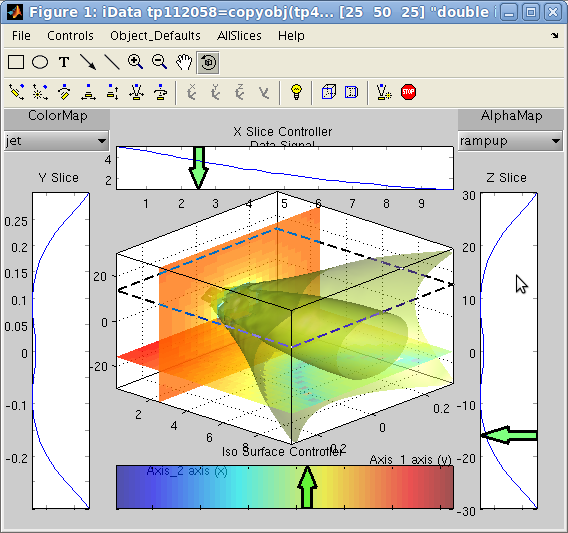The 'slice' method displays a plot rendering of a 2D and 3D objects. This interactive tool provides a powerful data set exploration interface. See details in the Plot help.

####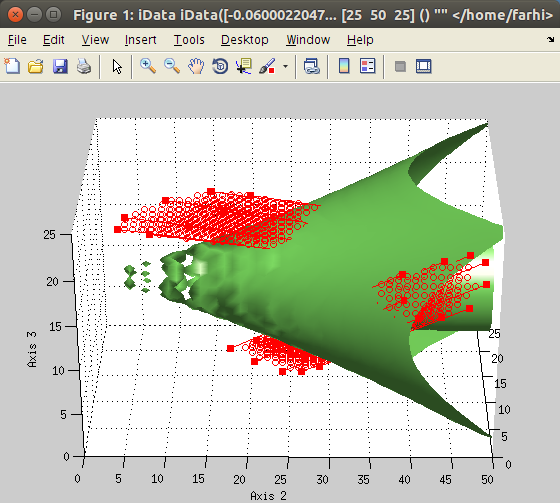Graphical user interface to select regions-of-interest (ROI)

The imroi method displays a data set and allows to define interactively polygon areas used to select portions of the initial data set. See details in the Plot help.

### Creating arrays of objects

The iData methods include a set of operators that generate arrays of objects. The result is either a array of empty objects, an array of constant objects, a linearly spaced array of objects, and a logarithmically spaced array of objects.

The zeros unary operator takes an iData object and a dimension as arguments. The result is an array made by duplicating the object.
`>> a = zeros(iData, [5 3]);    % creates a 5x3 empty iData array>> a = iData(peaks);>> b = zeros(a, 5, 3);         % creates a 5x3 constant array of 'peaks' (2D surface)`
The linspace and logspace binary operators create a linearly and logarithmically spaced array of objects, by gradually moving from one object to the other. This way, it may be considered as a 'morphing' operator.
`>> a = iData(peaks);>> b = linspace(a, cos(a), 5);>> c = logspace(a, sin(a), 5);>> subplot([b c],[2 5])`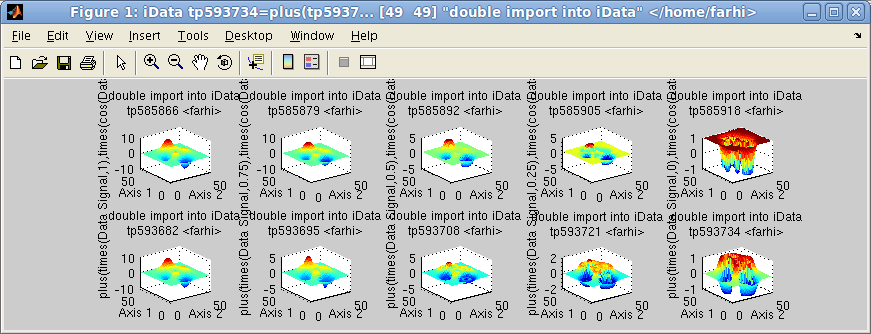Linspace (first row) and logspace (2nd row)

The colon operator a:b is the same as linspace with a number of steps which is the integer difference between the objects mean value.

### Interpolation, smoothing, intersection and union

Most binary operators require to interpolate the objects onto common axes prior to applying the mathematical operation. The common axes may be obtained from intersection or union of object axes.

The interpolation method used is based on a Delaunay triangular multidimensional tessellation (using the Computational Geometry Algorithms Library, CGAL).

#### Intersection and union

The intersect and union binary operators determine the minimum and maximum axes bounds, as well as a axes binning. The inner and outer values are returned by the intersect and union methods resp. Resulting objects are interpolated, using the interp operator, onto this new axis system, so that binary operators are easy to apply afterwards.
In the following example, we shift an object along axes, and signal value, and then show the intersection and union. Extrapolated values, for union, are set to 0.
`>> a = iData(peaks);>> b = copyobj(a);>> a{1} = a{1}+10; a{2} = a{2}+10;  % shift along X and Y>> a.Signal=a.Signal+5;             % shift along Z>> [ai,bi]=intersect(a,b);>> [au,bu]=union(a,b);>> subplot(1,3,1); plot([a  b ],'transparent tight')>> subplot(1,3,2); plot([ai bi],'transparent tight')>> subplot(1,3,3); plot([au bu],'transparent tight')`

#### Interpolation

The interp operator can be used to interpolate one object onto an other axis coordinate frame, which can be given as an other object, a binning scaling factor, or a set of axes. The interpolation method can be specified as an additional character argument amongst linear (default), cubic, spline, nearest.
`>> a = iData(peaks(10))+2>> b = interp(a,2)	% doubled binning, but we rather recommend the 'resize' method (see below)>> c = interp(a,1:.25:15,3:.25:12)>> subplot([a b c],[1 3],'mesh tight')`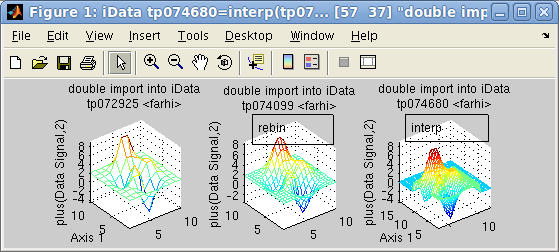#### Event data sets

It is possible to create an event data set from a column-type object (that is a matrix which columns are axes/data) by means of the 'event' method (which creates a cloud of points) or the 'hist' method (which creates an histogram out of an event list).

For event based data sets, the hist operator transforms the event object into an histogrammed data set. The histogram axes can be given as vectors (where event will be accumulated), or a number of bins within the [min max] of the distributions. The hist operator is much faster than the interpolation one, interp for event based data.
`>> a=iData([ ifitpath 'Data/Monitor_GV*']);>> b=hist(a);>> surf(b, 'median');`
If the number of events is limited compared to the number of bins in the histogram, some areas of the histogram can remain empty when filling it with the events. It is then possible to 'fill' the empty slots with a fast neighboring algorithm, using a discrete cosine transform.
`>> a=iData([ ifitpath 'Data/Monitor_GV*']);>> b=hist(a); >> c=fill(b);>> subplot(a,b,c);`

#### Re-binning and resizing

To compress an object and reduce its binning, that is the number of elements on each dimension, you can use the reducevolume method. Without argument, it reduces the number of elements to less than 1e6. Additional arguments indicate the compression factor on each dimension. This method is automatically used when plotting large objects (except if the plot options contains a 'full' or 'whole' word).

In a similar manner, the resize method is a very fast yet accurate algorithm (discrete cosine transform) to make a fast rebinning, that is change the number of elements on each dimension/rank, but preserve the data set meaning.
`>> a = iData(peaks(40))+2>> subplot(a, resize(a, [20 20]), resize(a, 40, 40)) % resize operation`
This allows for instance to quickly reduce the dimensionality of a data set:
`>> b = iData(flow);>> resize(b, [25 50 1]);	% makes a 2D object from a 3D one`
Reference: Narasimha M. et al, On the computation of the discrete cosine transform, IEEE Trans Comm, 26, 6, 1978, pp 934-936.

#### Smoothing

To explicitly smooth a data set, you can use the 'smooth' method, which makes use of a discrete cosine transform filter spline smoothing (shape preserving):
`>> a = iData(peaks(40).*(1+0.1*randn(40)));>> b = smooth(a);>> subplot(a,b);`
Reference: Garcia D, Robust smoothing of gridded data in one and higher dimensions with missing values. Computational Statistics & Data Analysis, 2010.

The smoothing can also use a Savitsky-Golay moving average, but may lead to significant sharp features modifications (be cautious).
`>> b = smooth(a, 'sgolay');>> b = smooth(a, span, 'sgolay', degree); % Savitsky Golay degree and span (length) specified`
Last, you can use a nD Gaussian blur
`>> b = smooth(a, 'gauss',1);		% Gaussian smoothing, all width=1>> b = smooth(a, 'gauss',[ 1 10 ]);	% idem, with specific width, here for a 2D smoothing`
Last, in the case a data set contains NaN (Not-a-Number) values (that is missing values), you can fill these values with a fast interpolation on neighboring elements using the fill method (based on a discrete cosine transform).

#### Manipulating object dimensions

It is also possible to manipulate an object Signal in the same way as a matrix with methods reshape (re-order elements in the object), permute (permute ranks, that is a generalized transposition) and circshift (shift ranks/dimensions), which work just as their matrix equivalent.

Last, the sort operator sorts object axes, in ascending or descending order. The corresponding Signal, Error, and Monitor values are also re-ordered accordingly.

E. Farhi - iFit/Math operators -  Nov. 27, 2018 2.0.2 - back to Main iFit Page